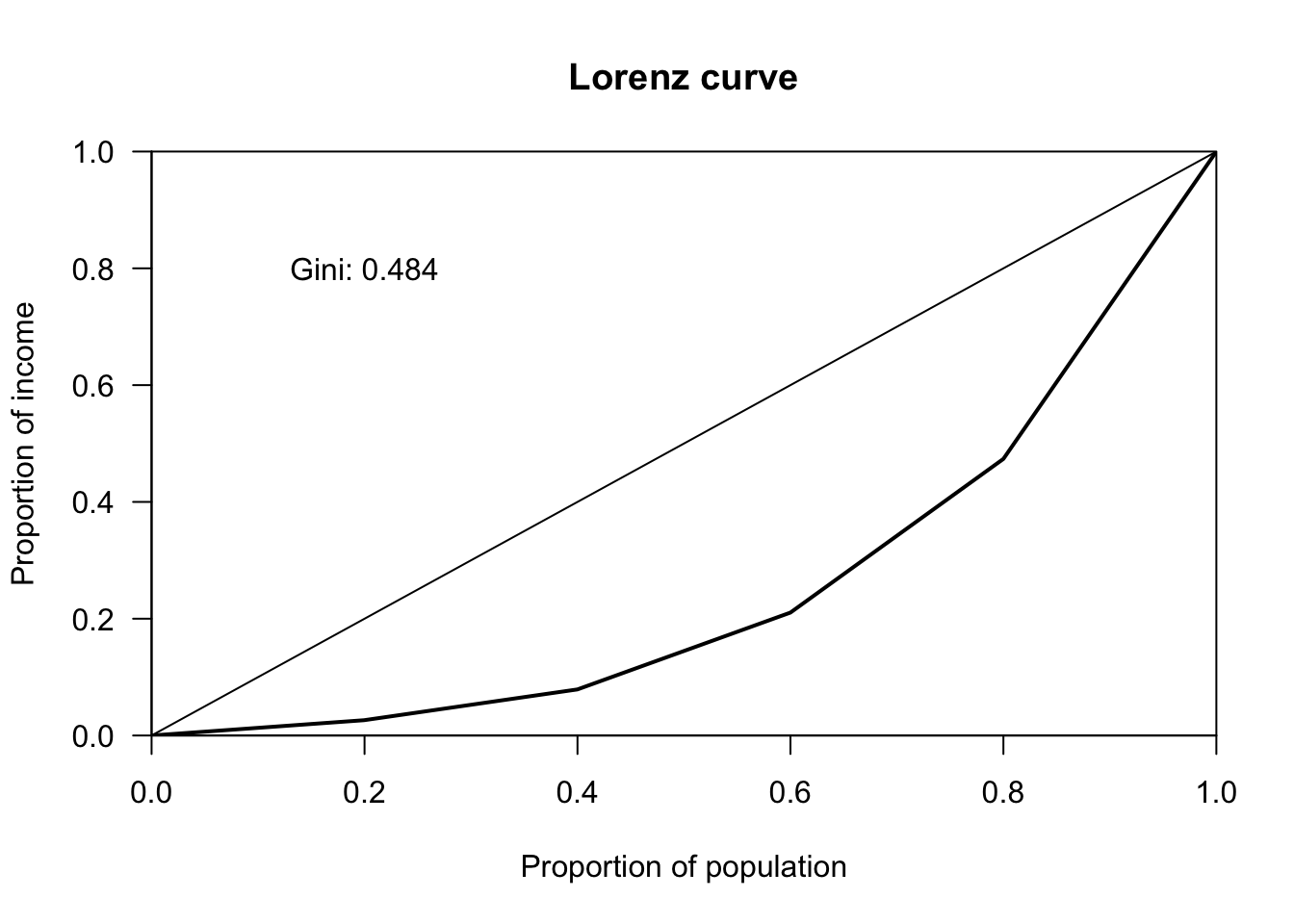# The firm I

Materials for class on Tuesday, February 19, 2019

## Calculating Gini coefficients

You can see global inequality statistics at Wikipedia. There are several columns here—the World Bank and CIA both calculate Gini coefficients (multiplied by 100 to be on a 0–100 scale), and the R/P columns are the 90/10 ratios you calculated in Problem Set 1 (income held by top 10% compared to income held by bottom 10%).

Here’s an example of how to calculate Gini coefficients in Excel:

Instead of using calculus to find the area under Lorenz curve, we used this formula where $$x$$ = income, $$y$$ = cumulative proportion of the population, and $$\mu_x$$ = mean of income:

$\text{Gini} = \frac{2}{\mu_x} \text{Cov}(x, y)$

You can also do this in R with the ineq package:

library(ineq)

# List of incomes
incomes <- c(10000, 20000, 50000, 100000, 200000)

# Calculate Gini coefficient
gini_coef <- Gini(incomes)
gini_coef
##  0.4842105
# Plot Lorenz curve
plot(Lc(incomes), xlab = "Proportion of population", ylab = "Proportion of income")
text(x = 0.2, y = 0.8, labels = paste("Gini:", round(gini_coef, 3)))## Market for lemons

This is pretty exciting, since the experiment worked perfectly! This game comes from experiment 9 by Dirk Yandell, Using Economic Experiments in the Classroom (Upper Saddle River, New Jersey: Prentice Hall, 1999). The book is sadly out of print and doesn’t exist anywhere, but an abstract of the game is listed as Game #66 here.

In the first round, lemons dominated and few good cars were sold. Buyers who got the lemons lost thousands of dollars, while the sellers walked away with phenomenal profits. Had we kept repeating the simulation under these rules, the good cars would have evaporated completely and the market would have been dominated by lemons.

In the second round, though, given the opportunity to buy the right to share information (sellers could pay \$200 for the ability to advertise the quality of their cars), good cars returned and lemons almost disappeared completely.

## Clearest and muddiest things

Go to this form and answer these three questions:

1. What was the muddiest thing from class today? What are you still wondering about?
2. What was the clearest thing from class today?
3. What was the most exciting thing you learned?

I’ll compile the questions and send out answers after class.